Courses

# Trigonometry Identities, Applications - Introduction to Trigonometry, Class 10, Mathematics Class 10 Notes | EduRev

## Class 10: Trigonometry Identities, Applications - Introduction to Trigonometry, Class 10, Mathematics Class 10 Notes | EduRev

The document Trigonometry Identities, Applications - Introduction to Trigonometry, Class 10, Mathematics Class 10 Notes | EduRev is a part of Class 10 category.
All you need of Class 10 at this link: Class 10

T - IDENTITIES (Trigonometry Identities)

We know that an equation is called an identity when it is true for all values of the variables involved. Similarly, an equation involving trigonometric ratios of an angle is called a trigonometric identity, if it is true for all values of the angle(s) involved.
The three Fundamental Trigonometric Identities are –

(i) cos2 A + sin2 A = 1 ; 0� ≤ A ≤ 90�
(ii) 1 + tan2 A = sec2 A ; 0� ≤ A < 90�
(iii) 1+ cot2 A = cosec2 A; 0� < A ≤ 90�

Geometrical Proof : Consider a ΔABC, right angled at B. Then we have :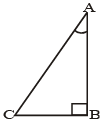AB2 + BC2 = AC2 ...(i) By Pythagoras theorem

(i) cos2 A + sin2 A = 1 ; 0� ≤ A ≤ 90� Dividing each term of (i) by AC2, we get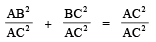i.e.,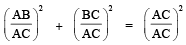i.e., (cos A)+ (sin A)2 = 1 i.e., cos2 A + sin2 A = 1 ...(ii)

This is true for all A such that 0� ≤ A ≤ 90�
So, this is a trigonometric identity.
(ii) 1 + tan2 A = sec2 A ; 0� ≤ A < 90� Let us now divide (i) by AB2. We get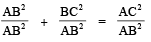i.e.,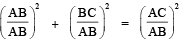i.e., 1 + tan2 A = sec2A ...(iii)

This equation is true for A = 0�. Since tan A and sec A are not defined for A = 90�, so (iii) is true for all A such that 0� ≤ A < 90�

(iii) 1 + cotA = cosec2 A ; 0� < A ≤ 90�

Again, let us divide (i) by BC2, we get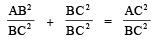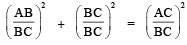⇒ 1 + cot2 A = cosec2 A ...(iv)

Since cosec A and cot A are not defined for A = 0�, therefore

(iv) is true for all A such that  0� < A ≤ 90� Using the above trigonometric identities, we can express each trigonometric ratio in terms of the other trigonometric ratios, i.e., if any one of the ratios is known, we can also determine the values of other trigonometric ratios.

Fundamental Identities (Results)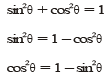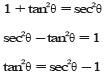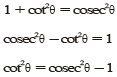To prove Trigonometrical Identities The following methods are to be followed :

Method-I: Take the more complicated side of the identity (L.H.S. or R.H.S. as the case may be) and by using suitable trigonometric and algebraic formulae prove it equal to the other side.

Method-II : When neither side of the identity is in a simple form, simplify the L.H.S. and R.H.S. separately by using suitable formulae (by expressing all the T-ratios occuring in the identity in terms of the sine and cosine and show that the results are equal).

Method-III : If the identity to be proved is true, transposing so as to get similar terms on the same side, or if cross-multiplication, and using suitable formulae, we get an identity which is true.

Ex.17 Express sin A, sec A and tan A in terms of cot A.

Sol. We know that

sin A =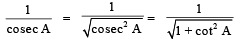sec A =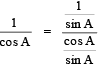...(Dividing num. and deno. by sin A)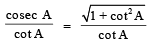and tan A =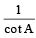Ex.18 Prove

Sol. L H S =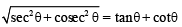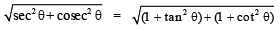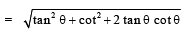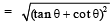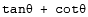= RHS

Hence, proved.

Ex.19 Prove (cosecA – sinA) (sec A – cos A)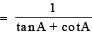Sol. LHS = (cosecA – sinA) (sec A – cos A) =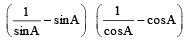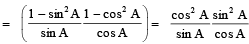[∵ sin2 A + cosA = 1]

= sin A cos A =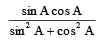[∵ sin2 A + cos2 A = 1]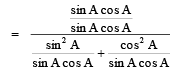[Dividing the numerator and denominator by sin A cos A.]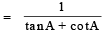Hence, proved.

APPLICATIONS OF TRIGONOMETRY

Many times, we have to find the height and distances of many objects in real life. We use trigonometry to solve problems, such as finding the height of a tower, height of a flagmast, distance between two objects, where measuring directly is trouble, some and some times impossible. In those cases, we adopt indirect methods which involves solution of right triangles.
Thus Trigonometry is very useful in geography, astronomy and navigation. It helps us to prepare maps, determine the position of a landmass in relation to the longitudes and latitudes. Surveyors have made use of this knowledge since ages.
Angle of Elevation The angle between the horizontal line drawn through the observer eye and line joining the eye to

any object is called the angle of elevation of the object, if the object is at a higher level than the eye i.e., If a horizontal line OX is drawn through O, the eye of the observer, and P is an object in the vertical plane through OX, then if P is above OX, as in fig. ∠XOP is called the angle of elevation or the altitude of P as seen from O.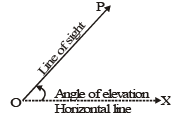Angle of Depression The angle between the horizontal line drawn through the observer eye and the line joining the eye to any object is called the angle of depression of the object if the object is at a lower level than the eye. i.e., lf a horizontal line OX is drawn through O, the eye of an observer, and P is an object in the vertical plane through OX, then if P is below OX, as in fig. ∠XOP is called the angle of depression of P as seen from O.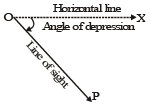REMARK :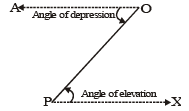1. The angle of elevation as well as angle of depression are measured with reference to horizontal line.
2. All objects such as towers, trees, mountains etc. shall be considered as linear for mathematical convenience,throughout this section.
3. The height of the observer, is neglected, if it is not given in the problem.
4. Angle of depression of P as seen from O is equal to the angle of elevation of O, as seen from P.i.e., ∠AOP = ∠OPX.
5. To find one side of a right angled triangle when another side and an acute angle are given, the hypotenusealso being regarded as a side.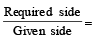a certain T-ratio of the given angle.
6. The angle of elevation increases as the object moves towards the right of the line of sight.
7. The angle of depression increases as the object moves towards the left of the line of sight.

COMPETITION WINDOW

BEARING OF A POINT

The true bearing to a point is the angle measured in degrees in a clockwise direction from the north line.
We will refer to the true bearing simply as the bearing.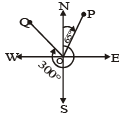e.g. (i) the bearing of point P is 65� (ii) the bearing of point Q is 300� A bearing is used to represent the direction of one point relative to another point. e.g., the bearing of A from B is 60�. The bearing of B from A is 240�.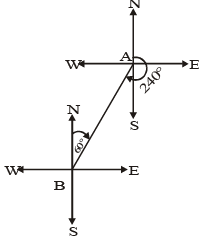TRY OUT THE FOLLOWING

State the bearing of the point P in each of the following diagrams :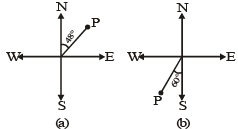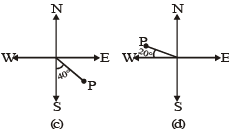(a) 48�  (b) 240� (c) 140� (d) 290� Ex.20  An observer 1.5 m tall, is 28.5 m away from a tower 30 m high. Determine the angle of elevation of the top of the tower from his eye.

Sol. Let AB be the height of the tower, CD the height of the observer with his eye at the point D, AB = 30 m, CD = 1.5 m.
Through D, draw DE II CA then ∠BDE = q where q is the angle of elevation of the top of the tower from

his eye. AC = horizontal distance between the tower and the observer = 28.5 m BE = AB - AE = (30 - 1.5) m = 28.5 m BDE is right triangle at E,
then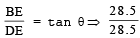= tan θ ⇒ tan θ = 1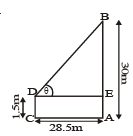⇒ tan θ = 1 = tan 45� ⇒ θ = 45�.  Required angle of elevation of the tower = θ = 45�.

Ex.23 A captain of an aeroplane flying at an altitude of 1000 metres sights two ships as shown in the figure. If the angle of depressions are 60� and 30�, find the distance between the ships.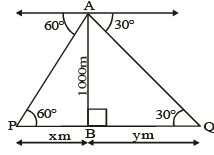Sol. Let A be the position of the captain of an aeroplane flying at the altitude of 1000 metres from the ground.
AB = the altitude of the aerplane from the ground = 1000 m P and Q be the position of two ships.
Let PB = x metres, and BQ = y metres.
Required : PQ = Distance between the ships = (x + y) metres.
ABP is rt. Δ at B ABQ is rt. Δ at B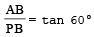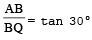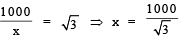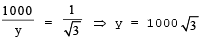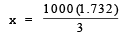577.3 my = 1000 (1.732) = 1732 m

Required distance between the ships = (x + y) metres = (577.3 + 1732) m = 2309.3 m

Ex.24 Two poles of equal heights are standing opposite to each other on either side of a road, which is 80 metres wide. From a point between them on the road, the angles of elevation of their top are 30� and 60�. Find the position of the point and also the height of the poles.
Sol. Let AB and CD be two poles of equal height standing opposite to each other on either side of the road BD. ⇒ AB = CD = h metres.
Let P be the observation point on the road BD. The angles of elevation of their top are 30� and 60�. ∠APB = 30�, ∠CPD = 60�
The width of the road = BD = 80 m, let PD = x metres Then BP = (80 – x) metres consider right DCDP, we have :
h II I h 30� 60�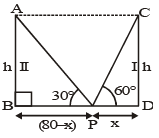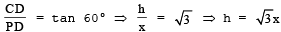...(i)
In right ΔABP, we have : AB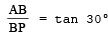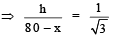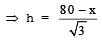...(ii)
From (i) and (ii), we get :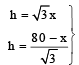⇒ (80 – x) = 3x ⇒ 4x = 80 ⇒ x = 20
Height of each pole = AB = CD =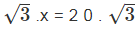= 20 (1.732) = 34.64 metres.
Position of point P is 20 m from the first and 60 m from the second pole. i.e., the position of the point P is 20 m from either of the poles.

The document Trigonometry Identities, Applications - Introduction to Trigonometry, Class 10, Mathematics Class 10 Notes | EduRev is a part of Class 10 category.
All you need of Class 10 at this link: Class 10Use Code STAYHOME200 and get INR 200 additional OFF Use Coupon Code
All Tests, Videos & Notes of Class 10: Class 10

### Top Courses for Class 10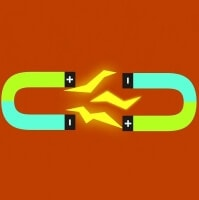### Top Courses for Class 10Track your progress, build streaks, highlight & save important lessons and more!

,

,

,

,

,

,

,

,

,

,

,

,

,

,

,

,

,

,

,

,

,

,

,

,

,

,

,

,

,

,

;